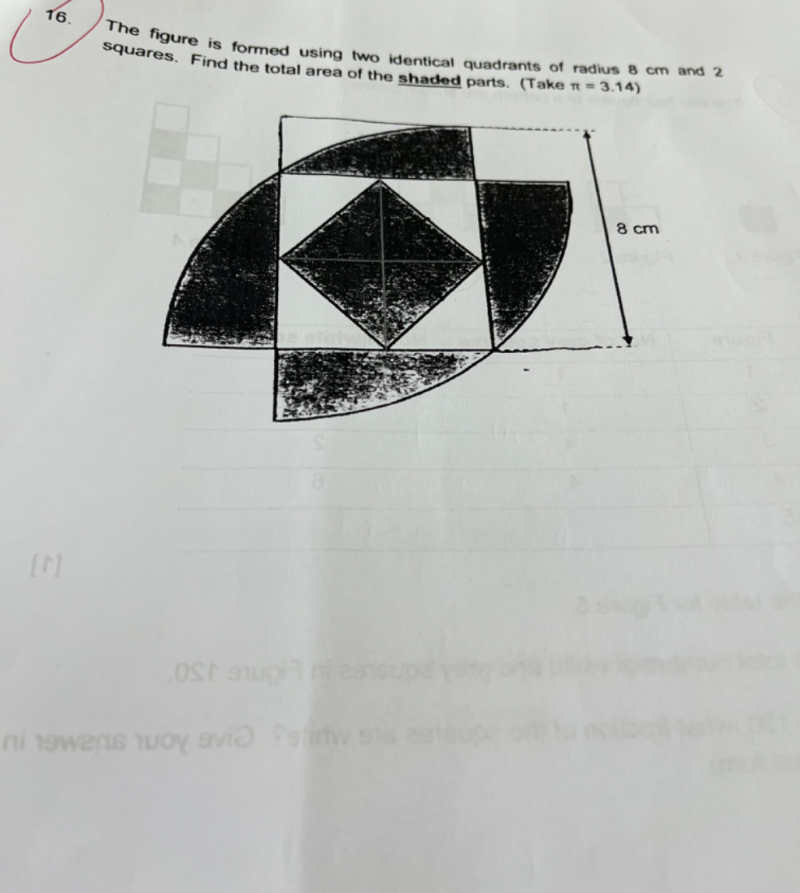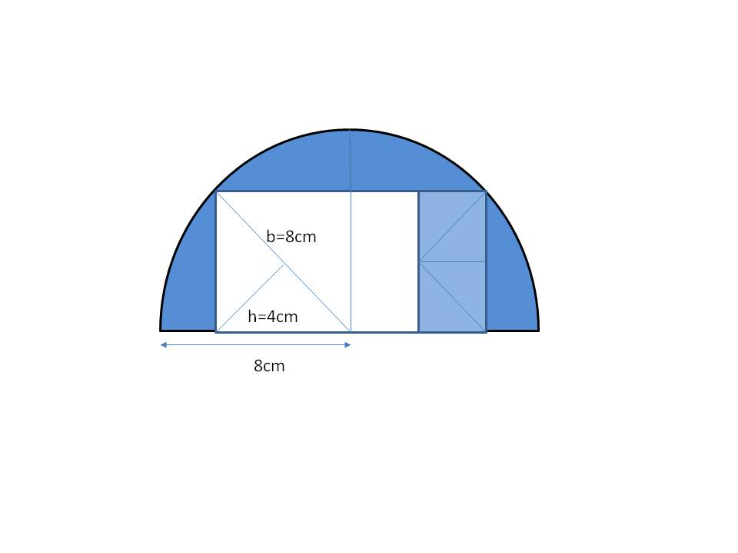# QuestionHi, anyone can help?If you pull out the 2 quadrants and put them side by side, the shaded small square will become 1/4 of the rectangle(2 big squares)

The shaded portion of the original diagram = The blue parts of this new diagram

Area of semi-circle = πr2 /2 = (3.14 x 8 x 8) / 2 = 100.48

Area of triangle = ( 1/2) b x h = 1/2 x 8 x 4 = 16 = Area of the 4 blue small triangles forming a rect.

Since the white part is made of 3 times of the blue rectangle,  area = 3 x 16 = 48

Hence area of shaded part = Area of semi circle – Area of white part = 100.48 – 48 = 52.48 cm2